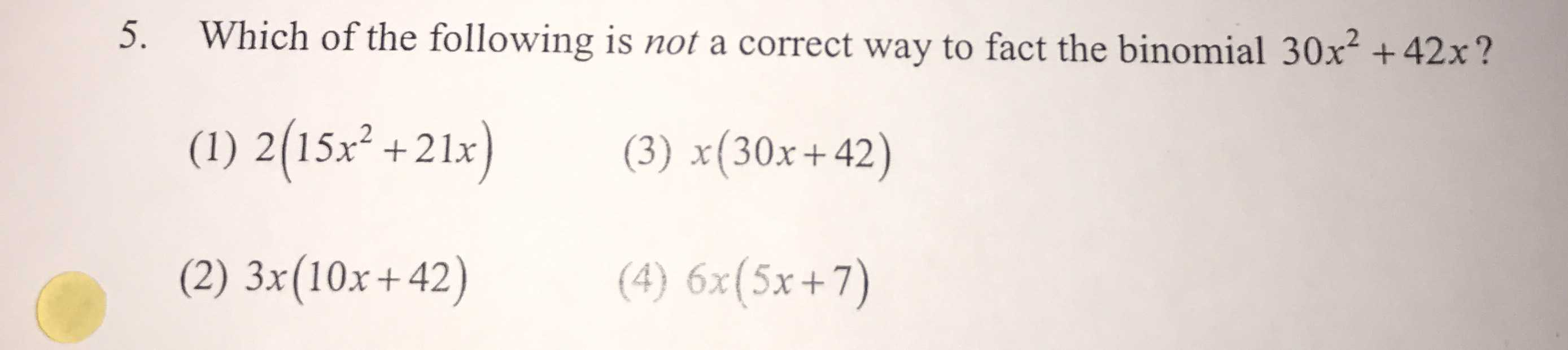### ¿Todavía tienes preguntas de matemáticas?

Pregunte a nuestros tutores expertos
Algebra
Pregunta5. Which of the following is not a correct way to fact the binomial $$30 x ^ { 2 } + 42 x$$ ?

(1) $$2 ( 15 x ^ { 2 } + 21 x )$$ (3) $$x ( 30 x + 42 )$$

(2) $$3 x ( 10 x + 42 )$$ (4) $$6 x ( 5 x + 7 )$$